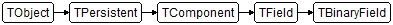# API:Data.DB.TBinaryField

Jump to: navigation, searchDelphi

```TBinaryField = class(TField)
```

C++

```class PASCALIMPLEMENTATION TBinaryField : public TField
```

## Contents

#### Properties

Type Visibility Source Unit Parent
class public
Data.DB.pas
Data.DB.hpp
Data.DB Data.DB

## Description

TField is the common ancestor of all the field components.

Data.DB.TBinaryField inherits from Data.DB.TField. All content below this line refers to Data.DB.TField.

TField is the common ancestor of all the field components.

TField encapsulates the fundamental behavior common to all field components. It introduces the properties, events, and methods that are used to:

• Change the value of a field in a dataset.
• Convert the value of a field from one data type to another.
• Validate data that the user enters for a field.
• Define how the data in the field appears as it is displayed or edited.
• Calculate the value of a field from code written in the OnCalcFields event of the dataset.
• Look up the field's value from another dataset.

Do not create instances of TField. TField descendants are created automatically each time a dataset is activated. These descendants can be dynamic (the default) or persistent. Dynamic field components reflect the columns in the underlying metadata at the time a dataset is opened. Persistent field components are created at design time using the Fields editor, which specifies the fields in the dataset, their properties, and their ordering.

Creating persistent field components guarantees that each time an application runs, it uses and displays the same columns, in the same order, even if the physical structure of the underlying database changes. If a column on which a persistent field component is based is deleted or changed, the IDE generates an exception rather than opening the dataset against a nonexistent column or mismatched data. If this happens, remove the field component for the nonexistent field using the Fields editor.

### Reading the Value of a TField Object

Use the DataType property to find out which type of value a TField object contains.

Use one of the properties prefixed with `As` to obtain the value of the TField object using a suitable type:

If you read a property that is not suitable for the type of your TField object, an exception raises.

If your TField object contains a null value, IsNull is `True`. When you read the value of a TField object that contains a null value, you obtain the default value of the corresponding type; for example, AsInteger is 0 for a null value.

### Subclasses

A field in a dataset is always treated as one of the following TField descendant classes:

Descendant class Description

A TADTField object represents an ADT (Abstract Data Type) field in a dataset.

TDateField represents a date field in a dataset.

TReferenceField represents a REF field in a dataset.

TAggregate field represents a maintained aggregate in a client dataset.

TDateTimeField represents a date-time field in a dataset.

Represents a signed 16-bit integer field in a dataset.

TArrayField represents an Array field in a dataset.

TFloatField represents a field that contains floating-point values in a dataset.

TSQLTimeStampField represents a date-time field in a DBExpress dataset.

TAutoIncField is a persistent field object for an autoincrement field in a dataset.

TFMTBCDField represents a binary-coded decimal (BCD) field in a dataset.

TStringField represents a string field in a dataset.

TBCDField represents a binary-coded decimal (BCD) field in a dataset.

TGraphicField represents a graphics field in a dataset.

TTimeField represents a time field in a dataset.

TBinaryField represents an untyped binary field in a dataset.

TGuidField represents a guid field in a dataset.

TVarBytesField represents a variable-length untyped binary field in a dataset.

TBlobField represents a field in a dataset that holds a reference to a binary large object (BLOB).

TIDispatchField represents an IDispatch field in a dataset.

TVariantField represents a variant field in a dataset.

TBooleanField represents a field containing Boolean values.

Represents a signed 32-bit integer field in a dataset.

TWideStringField represents a large string field in a dataset.

TBytesField represents a bytes field in a dataset.

TInterfaceField represents an interface field in a dataset.

Represents an unsigned 16-bit integer field in a dataset.

TCurrencyField represents a field that contains currency values in a dataset.

A TLargeintField object represents a large integer field in a dataset.

TDataSetField provides access to nested datasets.

TMemoField represents a memo field in a dataset.